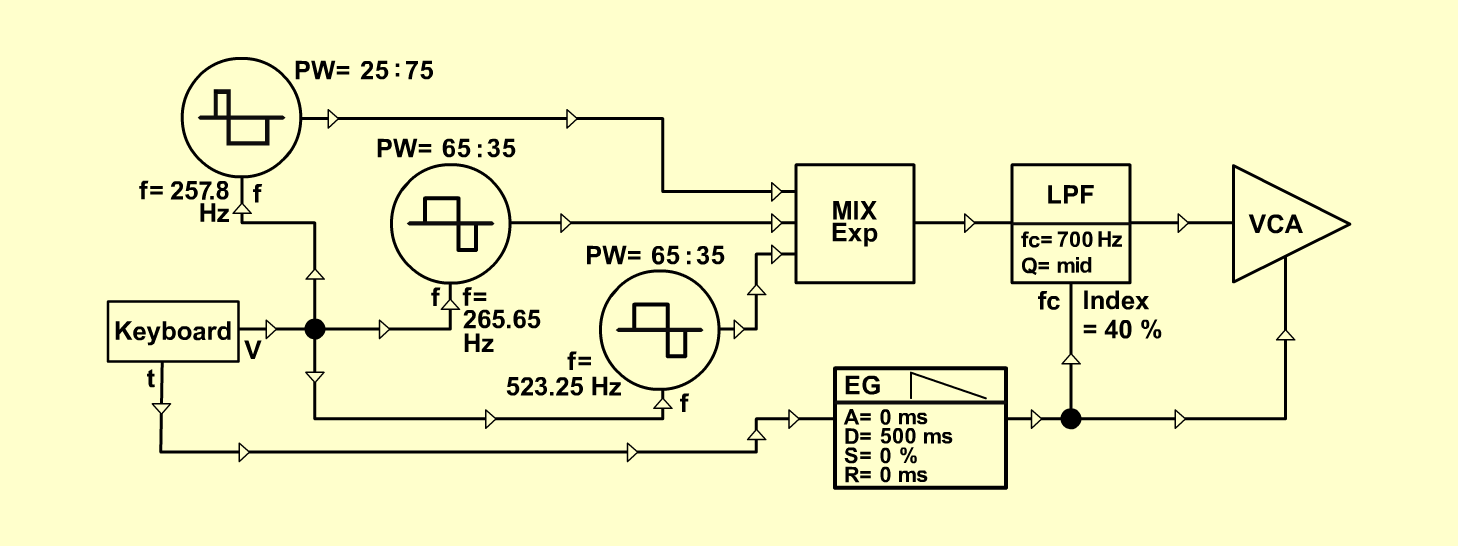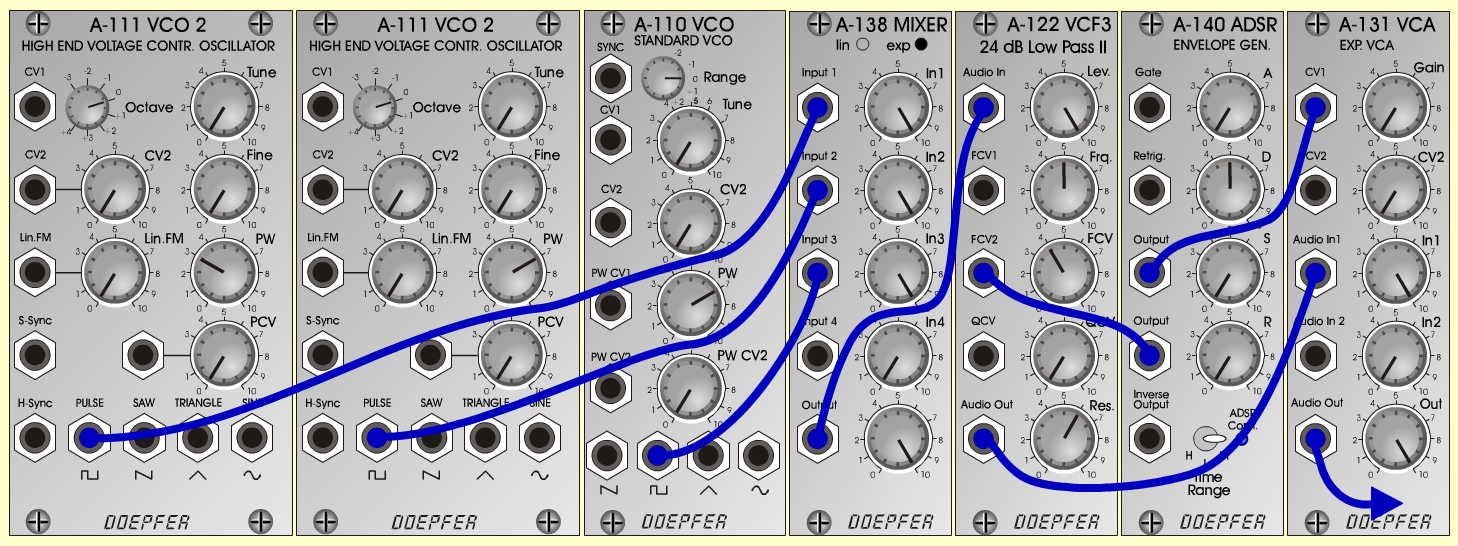Steel DrumsInstrumentsD o e p f e r A - 100 Connections: Settings: A-111/1 (Pulse)  <=>  A-138exp (Input 1) A-111/2 (Pulse)  <=>  A-138exp (Input 2) A-110 (Pulse)  <=>  A-138exp (Input 3) A-138exp (Output)  <=>  A-122 (Audio In) A-122 (Audio Out)  <=>  A-131 (Audio In 1) A-140 (Output/1)  <=>  A-122 (FCV 2) A-140 (Output/2)  <=>  A-131 (CV 1) KB (Pitch)  <=>  A-111/1+2 (CV1) KB (Pitch)  <=>  A-110 (CV1) KB (Gate)  <=>  A-140 (Gate) A-111/1 (PW = 3) A-111/2 (PW = 7) A-110 (PW = 7) A-122 (Level = 10, Freq = 5, FCV = 4, Res = 6) A-131 (Gain = 0, Audio In 1 = 10,  Audio Out = 10) A-138 (In 1 = 10, In 2 = 10, In 3 = 10, Out = 10) A-140 (A = 0, D = 5, S = 0, R = 0, Range = M) For Steel Dry: A-122 (Freq = 4) Notes: Tuning (e.g. 261,63 Hz):   A-110: one octave higher (e.g. 523,25 Hz)   A-111/1: 26 cents lower (e.g. 257,8 Hz)   A-111/2: 26 cents higher (e.g. 265,65 Hz) Josef MuellerSound samples Steel Drums (174 Hz) Steel Drums (349 Hz) Steel Drums (698 Hz) Steel Drums dry (174 Hz) Steel Drums dry (349 Hz) Steel Drums dry (698 Hz)